# GRE Subject Test: Chemistry : Covalent Bonding

## Example Questions

### Example Question #241 : Organic Concepts

The degree of unsaturation for ephedrine (shown below) is __________.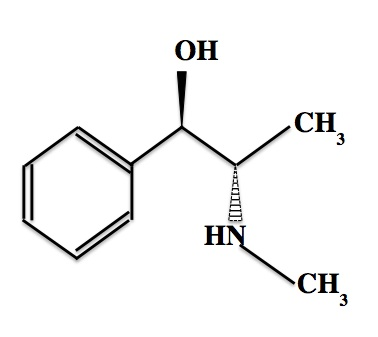Explanation:

The degree of unsaturation is equal to the number of rings plus the number of pi bonds in a molecule. Ephedrine has one ring and three pi bonds, so its degree of unsaturation is four.

To arrive at this answer, one could also use the formula below, whereis the number of carbon atoms,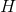is the number of hydrogen atoms,is the number of halogen atoms, andis the number of nitrogen atoms.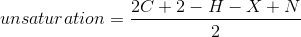For ephedrine,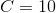,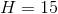,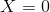, and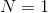.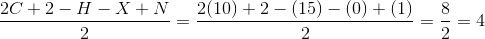### Example Question #1531 : Mcat Biological Sciences

2-butyne contains all of the following types of bonds except __________.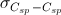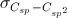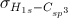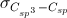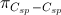Explanation:

2-butyne has the following chemical structure.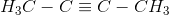The end carbons havehybridization (form single bonds only), while the middle two carbons have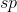hybridization (involved in a triple bond).  There are no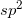hybridized carbons in this molecule.

### Example Question #1 : Covalent Bonding

For the compound shown below, the hybridization for carbon A is __________ and the hybridization for carbon B is __________.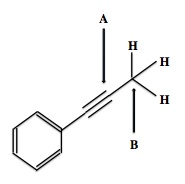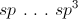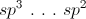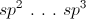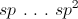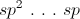Explanation:

Carbon A is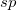hybridized because this carbon is only bonded to two other atoms. Carbon B is bonded to four atoms, and would therefore behybridized.

Keep in mind that a carbon involved in a triple bond will always behybridized, a carbon involved in a double bond will behybridized, and a carbon involved only in single bonds will be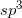hybridized.

### Example Question #1 : Compounds, Molecules, And Bonds

Which is not a property of covalent compounds?

They are poor electrical conductors

Many are not soluble in water, but are soluble in nonpolar liquids

They contain bonds formed by sharing one or more pairs of valence electrons between atoms

The elements involved generally have large differences in electronegativity

They have low melting and boiling points

The elements involved generally have large differences in electronegativity

Explanation:

The incorrect statement is a property of ionic compounds rather than covalent. Recall that electronegativity is a measure of the ability of an atom to draw electrons to itself. Ionic compounds are formed by elements with very different electronegativities, since elements with different electronegativities will tend to form positive and negative ions (that is, they give away or gain electrons easily). In contrast, covalent bonds are formed by elements which are close in electronegativity and could exist as stable free molecules. All other statements are true of covalent compounds.

### Example Question #11 : Compounds, Molecules, And Bonds

Which of the following molecules contains polar, covalent bonds?

I.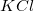II.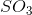III.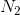IV.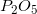V.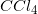II, IV, V

II, III, IV, V

III, IV, V

I, II, IV, V

II, IV

II, IV, V

Explanation: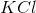is an ionic compound, whileis a nonpolar covalent compound. Remember that polarity results from a difference in the electronegativities of the atoms involved in the bond. Too great of a difference will result in an ionic bond; two of the same atoms will have zero difference, resulting in a nonpolar bond.,, andcontain polar covalent bonds. In the first two, oxygen will carry a slight negative charge, leaving sulfate and phosphorus with slight positive charges. In, chlorine will carry a slight negative charge, leaving the carbon slightly positive. In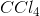and, symmetry helps to balance the polar bonds, resulting in an overall nonpolar molecule, even though the individual bonds are nonpolar.

### Example Question #11 : Bonds And Forces

Which of the following represents a triple bond?

Three pi bonds

Two sigma bonds and one pi bond

One sigma bond and two pi bonds

Three sigma bonds

Each of these combinations is possible

One sigma bond and two pi bonds

Explanation:

A sigma bond is a single covalent bond, involving an electron pair located between the two bonding atoms. A pi bond occurs when the p orbitals above and below the bonding atoms overlap, or when the p orbitals to the left and right overlap. In any covalent bond, the first bond formed is a sigma bond and any additional bonds must be pi bonds. Initial orbital overlap always comes from the sigma, or s, subshell; subsequent overlap comes from the pi, or p, subshells.

### Example Question #1 : Covalent Bonds

Which of the following molecules contains the shortest bond between nitrogen and oxygen?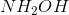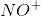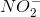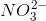Explanation:

This question is testing your ability to draw Lewis dot structures and your knowledge of how resonance effects bond length. The N-O bond with the greatest pi-bond character will be the shortest; thus, we are looking for a double- or triple-bond between nitrogen and oxygen.

Hydroxylamine () only contains single bonds, which have the least pi-bond character.

The nitrite and nitrate ions both have a double bond between the nitrogen and oxygen, but also one or more single bonds between these elements. This means that, because of resonance, the N-O bonds in these molecules will be averaged and our average bond order will be somewhere between single and double. Nitrate will have one double bond and two single bonds, for an average bond order of 1.33. Nitrite has one single bond and one double bond, for a bond order of 1.5.

The nitrosyl ion, however, will contain a triple bonds between the nitrogen and oxygen atoms, giving it the greatest pi-bond character. This bond will contain more energy and be shorter than the bonds in the other answer options.

### Example Question #11 : Bonds And Forces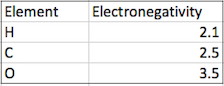Using the values from the given table, which single bond would be the most polar?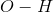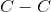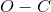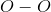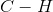Explanation:

Electronegativity of a chemical bond can be determined by calculating the electronegativity differences of the elements in a chemical bond. For example, using the values in the table given, the electronegativity differences between the bond are given below: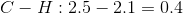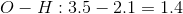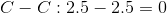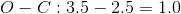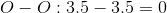Based on the calculations above, we can see that thebond has the highest difference and therefore has the highest electronegativity.

### All GRE Subject Test: Chemistry Resources